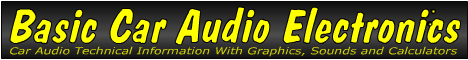Email   Home Page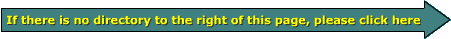Different Types of AC Voltage

3 voltages - 1 sine wave:
In one of the earlier examples, the power output of an amplifier was expressed as peak power. The voltage used to calculate the power output into the resistive load was peak voltage. The next section will explain the different ways to measure voltage.

Choices:
There are 3 ways to quantify the magnitude of a sine wave.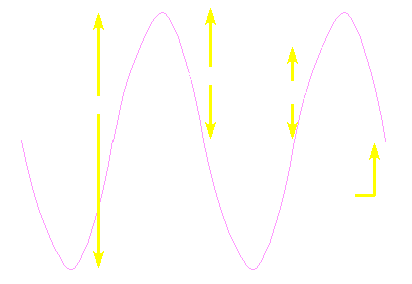Peak Voltage:
Peak voltage tells you how far the voltage swings, either positive or negative, from the point of reference. Peak voltage is only a moderately useful way of measuring voltage when trying to express the amount of work that will be done when driving a specified load. Some manufacturers use peak voltage to get the power output ratings of their amplifiers. I will explain this in more detail a little later.

Peak-Peak Voltage:
It is rarely used. It is probably more useful in the case of a non-symmetrical wave form. Otherwise you would probably express it's value as peak voltage.

RMS Voltage:
RMS voltage is absolutely the most common way to measure/quantify AC voltage. It is also the most useful. Because AC voltage is constantly changing and is at or near the highest and lowest points in the cycle for only a tiny fraction of the cycle, the peak voltage is not a good way to determine how much work can be done by an AC power source (e.g. your amplifier, a wall outlet in your house...). DC voltage is constant. Its voltage level can be plugged directly into the formulas for power (on the Ohm's law page) and you will get an accurate image of its ability to do work. RMS voltage will give you the same ability to predict how much work will be done by an AC voltage. The RMS voltage of a pureć sine wave is approximately .707*peak voltage. If you read voltage with a voltmeter you are generally given the RMS voltage of the wave form. Some meters display an 'average' voltage which is very close to RMS. When reading voltage with a voltmeter, the display indicates the RMS or average voltage not the peak or peak-peak voltage.

ćIf the waveform isn't a pure sine wave (like a square wave or a signal with mixed sine waves of different frequencies or music), multiplying the peak times .707 will not give an accurate RMS value and therefore will not give an accurate indication of the work that the waveform can produce when driving a load. For more complex signals, you need a meter that will calculate the RMS value from a set of samples taken at regular intervals. One such device (the TRUE RMS voltmeter) is discussed below.

More on RMS Voltage:
In the following diagram, the 'filled' area is where the sine wave will do some work (motivating speakers, etc.). The amount of work that it will do at any given point in time is determined by the magnitude (above and below the reference) of the voltage (with reference to the red line).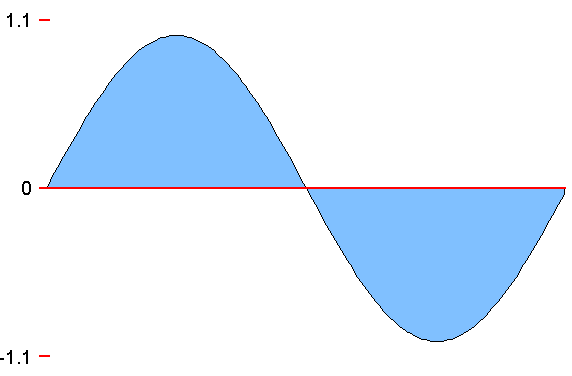If you look at the the blue area of the diagrams, this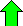is equal to this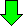. The 'negative' voltages were simply converted to positive voltages (for clarity). The green area is the equivalent DC voltage. As you can see in the diagram below, for the same period of time, the AC voltage is sometimes lower and sometimes higher than the DC voltage. The amount of work that can be done by the voltages in this diagram is the same. Of course when I say they are equal in the amount of work they can do, I am talking about something like heating a heating element or driving an electric motor and not audio reproduction (audio is an AC waveform). Note that the DC voltage is roughly equivalent to 71% of the peak AC voltage.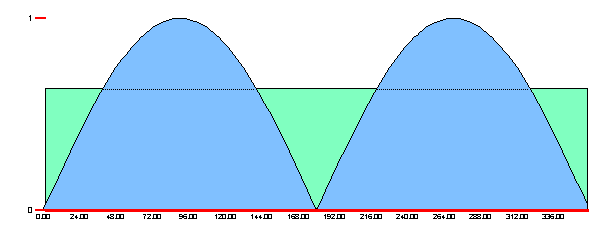Calculating Actual RMS Voltage:
If you have a 'true RMS' voltmeter, the meter measures the instantaneous voltage at regular time intervals. On the following graph, the little vertical lines along the sine wave represent the points in time where the voltage is measured. The microprocessor in the voltmeter then 'squares' all of the voltages at each point and adds the squared values together. It then calculates the average (mean) from the squared values. And finally... it calculates the square root of the average (mean) value.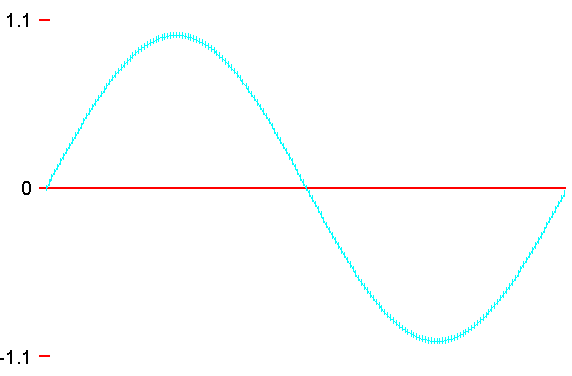The following is a sample of the data that's used to calculate the true RMS voltage of a 1 peak volt sine wave. The data is taken at 20║ intervals (about 1/10 the data points shown on the previous diagram). As you can see the values come out to the same value as when you multiply the voltage (1 volt in this case) by 0.707 (0.707 = 1/square root of 2).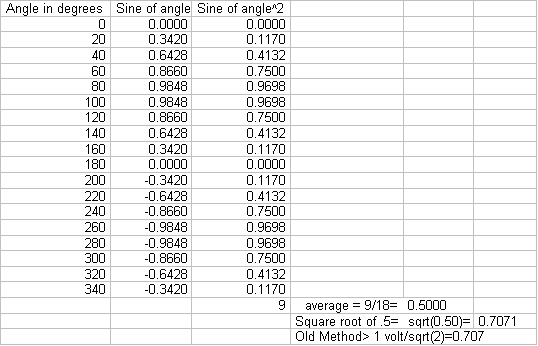Warning:
A word of caution, many manufacturers of car audio can exaggerate their power ratings by using peak power (derived by using peak voltages). Peak power is twice as much as RMS power and is not indicative of the actual amount of work that the amp will do (driving your speakers).

RMS & Continuous Power output

Confusion:
Many people wrongly believe that the RMS power rating is the same as a continuous power rating.

Power:
First, power is a 'snapshot' of the amount of work being done at any point in time. It has no specified time component.

Ratings:
There are a lot of different ways to rate an amplifier's output power capabilities.

A Few Ways to Rate Amplifier Output Power

• Watts
(not much information and lends itself to many different interpretations)

When manufacturers state the power output in 'watts' (and only 'watts' i.e. 50 watts), they want you to assume that the wattage rating is an accurate measurement of the amplifiers ability to do a given amount of work (driving your speakers). In reality, the power rating could be derived by using peak voltage instead of the more honest RMS voltage when plugging the numbers into the formula P=E^2/R. This would give a power output/rating that's TWICE the RMS power output. Actually they could be using virtually anything to derive this vague specification (pulling a number out of thin air comes to mind).

• Peak watts
(misleading because many will take it as a true measure of the work that the amplifier will do when driving the speakers)

If the manufacturer specifies wattage as 'peak power' they may say that they aren't trying to mislead their customers, but I believe that that is precisely what they are trying to do. They assume that the vast majority of people are going to accept the wattage as the maximum power that the amplifier can produce. If they use peak voltage to derive the peak power, the power specification may be legitimate but it is misleading. The peak power is mathematically twice the RMS power output.

• RMS watts
(Better than peak power but it also lends itself to misinterpretation because it contains no specified element of time)

RMS wattage is an accurate way to measure power but the amplifier may only be able to produce the RMS voltage into the given load for a fraction of a second. The, less than honest, manufacturer may plug this RMS voltage into the P=E^2/R formula and give this number as the amplifier's output. If they give this as the power output of the amplifier but don't tell you that the amplifier can't continuously produce this power level, they are again misleading you.

• RMS continuous watts
(the best, most accurate, honest way to measure power output)

When manufacturers rate their amplifiers at a given number of RMS watts continuous power output. They are using RMS wattage (derived from RMS voltage) which is the most accurate way to state the useful power produced by the amplifier. There is only one way to interpret this. They are also stating that the amplifier can produce the power continuously. If they didn't specify continuous power they may be trying to cover up the fact that the amplifier could only produce the RMS voltage required to drive the given load to a given RMS power for a fraction of a second (like in the previous example). When they state continuous output power, they are saying that the amplifier can easily and continuously produce the rated output power.

For the Purists...

RMS Power (PRMS):
Throughout this site I will use the term 'RMS power'. Technically, as far as I know, there is no such thing as 'RMS' power. This section will help clarify and define a few terms. I will define RMS power as the power that's calculated when using an RMS voltage or current into a resistive load. Other ways to quantify power...

Average power (Pave):
Average power is defined as the RMS voltage multiplied by the RMS current.

Peak power (Ppeak):
Peak power is defined as the peak voltage multiplied by the peak current.

You May Be Interested in My Other Sites
• This site was started for pages/information that didn't fit well on my other sites. It includes topics from backing up computer files to small engine repair to 3D graphics software to basic information on diabetes.
• This site introduces you to macro photography. Macro photography is nothing more than the photography of small objects. It can take quite a while to understand the limitations associated with this type of photography. Without help, people will struggle to get good images. Understanding what's possible and what's not possible makes the task much easier. If you need to photograph relatively small objects (6" in height/width down to a few thousandths of an inch), this site will help.
• If you're interested in air rifles, this site will introduce you to the types of rifles available and many of the things you'll need to know to shoot accurately. It also touches on field target competition. There are links to some of the better sites and forums as well as a collection of interactive demos.
• This site helps anyone new to computers and anyone with a basic understanding of computers with a desire to learn more about the internal components of a computer. If you have a computer that you'd like to upgrade but don't know where to start, this is a good site for you.
• This site is for those who want to begin racing karts but don't fully understand how the various parts work. It's mostly interactive demos that show how the various parts of the kart work.

•  You should remember: 1.Peak voltage tells you how far a voltage swings above or below a reference. 2.RMS voltage is the most commonly used. 3.RMS voltage is equal to peak voltage*.707. 4.Peak Power is mathematically twice RMS power.

```

```